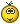# Big-eye killing shape

8 posts / 0 new
macelee
Big-eye killing shape

I am going through Japanese Meijin games in 1990's recently and found this very interesting game. How often can you see groups killed by Square Four? How about in a professional game? And how about two of them in the same game? That's exactly what happened in the game below:

Unfortunately I cannot include this game in Go4Go database for the moment because I cannot find the exact playing date. It is definitely played in 2003 and there is official record of this game in Nihon Kiin website without the date. Can you help?

mkeller
moves 124 to 134 were quite

moves 124 to 134 were quite amazing, will definitely have a closer look at those over the weekend

Lake
thanks for bringing up this

thanks for bringing up this game. seems b shouldn't have given w such an impressive wall.

meepanda
I can't see the diagram T_T

I can't see the diagram T_T

macelee
SGF

You must be using a tablet not supporting Flash. The SGF is below and you can copy/paste into your favourite SGF software.

(;
EV[19th Japanese Meijin, Preliminary]
DT[1993-??-??]
PB[Ishida Yoshio]BR[9p]
PW[Kudo Norio]WR[9p]
KM[5.5]RE[W+R]
;B[qe];W[dd];B[oq];W[dq];B[qp];W[od];B[pc];W[oc];B[pb];W[kc];B[do];W[co]
;B[dp];W[cp];B[eq];W[cn];B[dn];W[dm];B[cq];W[dr];B[bq];W[cr];B[cm];W[cl]
;B[br];W[er];B[bm];W[bn];B[fq];W[bp];B[bl];W[dl];B[ck];W[dk];B[cj];W[fo]
;B[ep];W[fr];B[gq];W[dj];B[ch];W[di];B[bg];W[dg];B[bs];W[aq];B[gr];W[ar]
;B[be];W[cf];B[bf];W[jp];B[gn];W[im];B[gl];W[hj];B[hk];W[ik];B[gj];W[gi]
;B[il];W[ij];B[jl];W[hm];B[gm];W[go];B[hl];W[en];B[ho];W[hp];B[gp];W[hn]
;B[io];W[ip];B[jo];W[ko];B[jm];W[el];B[kq];W[kp];B[ir];W[lq];B[lr];W[kr]
;B[jq];W[mr];B[lp];W[mq];B[mp];W[ln];B[jr];W[np];B[mo];W[op];B[no];W[pp]
;B[on];W[ll];B[ks];W[pq];B[qn];W[ms];B[ls];W[qq];B[rp];W[kk];B[or];W[os]
;B[nq];W[pr];B[nr];W[ns];B[rr];W[rq];B[sq];W[in];B[jn];W[fj];B[nl];W[mn]
;B[om];W[lj];B[ni];W[qm];B[pn];W[rn];B[qo];W[qj];B[qh];W[rh];B[rg];W[qi]
;B[ph];W[rl];B[jc];W[jb];B[ib];W[ic];B[jd];W[kb];B[dc];W[hb];B[ed];W[cd]
;B[cc];W[ef];B[gc];W[gb];B[fc];W[id];B[ri];W[rj];B[sh];W[pl];B[lm];W[mm]
;B[ml];W[km];B[mk];W[lk];B[mh];W[bd];B[bc];W[ob];B[me];W[pa];B[qb];W[lh]
;B[ff];W[fg];B[jf];W[jg];B[ee];W[ci];B[bi];W[cg];B[if];W[ig];B[gf];W[dh]
;B[bh];W[pd];B[qd];W[qa];B[ra];W[oa];B[pk];W[qk];B[rb];W[he];B[gg];W[gh]
;B[pm];W[sj]
)

goutte
move 107

Move 107 is amazing, no way I can see it in my games

pzykr
lots and lots of variations... how can one little corner have so many possible different ways to play? i guess that's why i'm still 10 kyu -- my opponents always seem to find one of those OTHER ways to play that i didn't think of